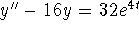# MA2051 - Ordinary Differential EquationsSample Exam #3 - B96

Second Exam - Originally Given 1996 A Term

Instructions: Do your work on the paper provided. Put your name, section number and PLA's name on that paper. Work neatly. Show your work. JUSTIFY YOUR ANSWERS.

1 45 pts
A spring is suspended from a fixed support as shown in the figure. In the coordinate system shown, an equation governing the motion of a mass m attached to this spring is my'' + ky = -mg. The lower end of this particular spring settles to rest at y = -0.098 m when a mass of 0.8 kg is hung from it.

1. Suppose a mass of 0.2 kg is suspended from the end of this spring. Write a model for the motion of the mass when it is started by lifting it up to y = 0.1 m and releasing it from rest.
2. Find the period of oscillation of the 0.2 kg mass. Would the period of oscillation of the 0.8 kg mass be larger or smaller?
3. The governing equation has a steady state. Find it and explain carefully why it is physically reasonable.
4. If the mass were immersed in a fluid, its oscillations would be damped, and the governing equation would have the form my'' + py' + ky = -mg. For what range of values of the damping coefficient p will the 0.2 kg mass oscillate? Would the 0.8 kg mass oscillate over the same range of p values?

2 30 pts
Consider the system1. Find its general solution.
2. Suppose y(0) = 2, z(0) = 4. Use one step of Euler's method to estimate y(3), z(3).

3 25 pts

1. Find a general solution of y'' - 16y = 0.
2. Find a general solution of.
3. Solve the initial-value problem, y(0) = y'(0) = 0.

```

© 1996 by Will Brother.
All rights Reserved. File last modified on December 6, 1996.

```